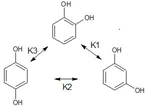Sample problems and solutions in Chemistry

Chemistry : Equilibrium constants, Enthalpy, Gibbs free energy, Arrhenius equation, rate constant, rate of reaction

Problem 1:  Consider a gas phase reaction involving the synthesis of ammonia from hydrogen and nitrogen at 350 oC

Starting with 1 mole of     and 3 moles of   , and no   to start with, Find

• An expression for the equilibrium constant in terms of the equilibrium conversion x
• If the equilibrium conversion at 298K and 1 atm is 0.6, find the equilibrium constant for the above reaction at 298K and 1 atm.

Problem 2For the gas phase reaction involving reactants X and Y, the individual heat capacities are 3 and 4 kcal/mol/K respectively while that of the products R and S are 6 and 7 kcal/mol/K respectively. Reactants X and Y react according to reaction below to form products P and Q at 227 oC and 5 atm . Find the heat of the reaction at 327 oC if the heat of the reaction at 227 oC is 100 kcal/mol.

Problem 3:  Isomerization of Catechol using hydrogen peroxide produces an equilibrium mixture of three isomers Catechol (ortho), Resorcinol (meta) and Hydroquinone (para) as represented by the scheme belowIf the initial concentration of pure catechol is Co , find the concentrations of the three isomers at equilibrium in terms  of the given constants. Make suitable assumptions if required.

Problem 4: Pyrolysis (thermal cracking) of large chain hydrocarbon n-decane into smaller hydrocarbons at 627 oC was found to have an activation energy of 32.86 kJ/mol and reaction rate r . If the same reaction was carried out at 927 oC, the reaction rate was found to be mr . Find m to the closest positive integer possible.

Chemistry   Set 1

Chemistry   Set 2

Chemistry   Set 3

Chemistry  Practice paper 1

Chemistry Practice Paper 2

Chemistry Practice paper 3

Chemistry Practice paper 4

Chemistry Practice Paper 5

Chemistry Practice Paper 6